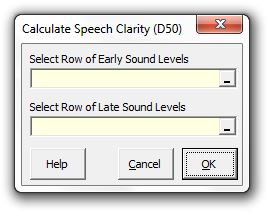### Strutt Help

Speech Clarity (D50)    1/1, 1/3

Strutt|Auditorium Acoustics|Speech Clarity (D50) calculates the objective speech clarity index D_(50) (definition, or Deutlichkeit) based on the early (0-50 ms) and late (>50 ms) components of the sound field, either measured or calculated using the Early Sound Level (Speech) and Late Sound Level (Speech) calculations.Strutt implements the calculation as:
D_50 = (int_0^50 p^2(t)dt)/(int_0^oo p^2(t)dt) = 10^(G_(0-50)/10)/10^(G_(0-oo)/10) i.e. D_50 is essentially the proportion of early energy in the sound field

Note that D_50 is related and convertible into to the vocal clarity index C_50 as follows:
C_50 = 10log(D_50/(1-C_50))